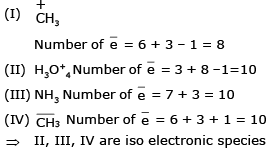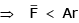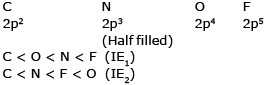Courses

# Classification Of Elements & Periodicity In Properties MCQ - 1 (Advance)

## 20 Questions MCQ Test Chemistry for JEE Advanced | Classification Of Elements & Periodicity In Properties MCQ - 1 (Advance)

Description
This mock test of Classification Of Elements & Periodicity In Properties MCQ - 1 (Advance) for JEE helps you for every JEE entrance exam. This contains 20 Multiple Choice Questions for JEE Classification Of Elements & Periodicity In Properties MCQ - 1 (Advance) (mcq) to study with solutions a complete question bank. The solved questions answers in this Classification Of Elements & Periodicity In Properties MCQ - 1 (Advance) quiz give you a good mix of easy questions and tough questions. JEE students definitely take this Classification Of Elements & Periodicity In Properties MCQ - 1 (Advance) exercise for a better result in the exam. You can find other Classification Of Elements & Periodicity In Properties MCQ - 1 (Advance) extra questions, long questions & short questions for JEE on EduRev as well by searching above.
QUESTION: 1

### Which of the following does not reflect the periodicity of element

Solution:

Neutron / proton ratio does not reflect the periodicity of element

QUESTION: 2

### Choose the s-block element in the following :

Solution:

The element receiving its last electron in its s-subshell according to (n + ℓ) rule is called s-block elemen

QUESTION: 3

### False statement for periodic classification of element is

Solution:

(f-subshell is filled)

QUESTION: 4

Pick out the isoleelctronic structure from the following :

I. +CH3

II. H3O+

III. NH3

IV. CH3-

Solution:

The chemical species having same number of electron in them are called isoelectronic speciesQUESTION: 5

The size of the following species increases in the order :

Solution:

for Inert gas Vander wall radius is definedQUESTION: 6

Element in which maximum ionization energy of following electronic configuration would be

Solution:

(On moving L to R in a period, I.E. increases

QUESTION: 7

The outermost electronic configuration of most electronegative element is :

Solution:

ns2, np5 belong to Halogen and they have larger value of EN in their respective periods

QUESTION: 8

The electron affinity of the member of oxygen of the periodic table, follows the sequence

Solution:

due to er EA of s > EA of O
(due to inter electronic repulsion in case of smaller 2p orbital of O)

QUESTION: 9

The process of requiring absorption of energy is

Solution:

ΔHeg2 is always endothermic

QUESTION: 10

In the following which configuration of element has maximum electronegativity.

Solution:
QUESTION: 11

Highest size will be of

Solution:
QUESTION: 12

Atomic radii of flourine and neon in Å units are respectively given by

Solution:
QUESTION: 13

The correct order of second ionisation potential of C, N, O and F is :

Solution:QUESTION: 14

Decreasing ionisation potential for K, Ca & Ba is

Solution:

As we move down the group size increases and the electrons experience less effective nuclear charge. Due to this reason, ionization potential decreases down the group. Thus, the second ionization potential of Ca is greater than that of Ba.

The second ionization potential of K is greater than that of Ca as K acquires stable noble gas configuration after losing one electron.

QUESTION: 15

Element Hg has two oxidation states Hg+1 & Hg+2. the right order of radii of these ions.

Solution:

QUESTION: 16

The ionization energy will be maximum for the process.

Solution:

(Lowest in size and highest Zeff.)

QUESTION: 17

Bond distance C - F in (CF4) & Si - F in (SiF4) are respective 1.33 Å & 1.54 Å. C - Si bond is 1.87 Å. Calculation the covalent radius of F atom ignoring the electronegativity differences.

Solution:
*Multiple options can be correct
QUESTION: 18

Two elements A & B are such that B. E of A – A, B – B & A – B are respectively 81 Kcal/mole, 64 Kcal/mole, 76 Kcal/ mole & if electronegativity of B is 2.4. then the electronegativity of A may be approxiamtely

Solution:
QUESTION: 19

Question No. 20 and 21 are based on the following information.
Four elements, P,Q,R & S have ground state electronic configuration as :
P → 1s2 2s2 2p6 3s2 3p3
Q → 1s2 2s2 2p6 3s2 3p1
R → 1s2 2s2 2p6 3s2 3p6 3d10 4s2 4p3
S → 1s2 2s2 2p6 3s2 3p6 3d10 4s2 4p1

Q. Comment which of the following option represent the correct order of true (T) & false (F) statement.

I. size of P < size of Q

II. size of R < size of S

III. size of P < size of R (appreciable difference)

IV. size of Q < size of S (appreciable difference)

Solution:

P is having atomic number 15 i.e. Phosphorous ( Group 15, Period 3)

Q is having atomis number 13 i.e. Aluminium (Group 13, Period 3)

R is having atomic number 23 i.e. Vanadium (Group 5. Period 4)

S is having atomic number 21 i.e. Scandium ( Group 3, Period 4)

Along the period atomic size decreases and down the group atomic size increases.

QUESTION: 20

Four elements, P,Q,R & S have ground state electronic configuration as :
P → 1s2 2s2 2p6 3s2 3p3
Q → 1s2 2s2 2p6 3s2 3p1
R → 1s2 2s2 2p6 3s2 3p6 3d10 4s2 4p3
S → 1s2 2s2 2p6 3s2 3p6 3d10 4s2 4p1

Q. Order of IE1 values among the following is

Solution: